Thermodynamics and Propulsion

# 8.7 Combined Cycles in Stationary Gas Turbine for Power Production

The turbine entry temperature in a gas turbine (Brayton) cycle is considerably higher than the peak steam temperature. Depending on the compression ratio of the gas turbine, the turbine exhaust temperature may be high enough to permit efficient generation of steam using the waste heat'' from the gas turbine. A configuration such as this is known as a gas turbine-steam combined cycle power plant. The cycle is illustrated in Figure 8.18.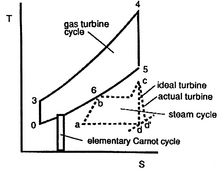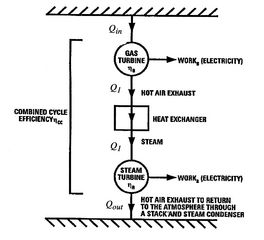The heat input to the combined cycle is the same as that for the gas turbine, but the work output is larger (by the work of the Rankine cycle steam turbine). A schematic of the overall heat engine, which can be thought of as composed of an upper and a lower heat engine in series, is given in Figure 8.19. The upper engine is the gas turbine (Brayton cycle) which expels heat to the lower engine, the steam turbine (Rankine cycle).

The overall efficiency of the combined cycle can be derived as follows. We denote the heat received by the gas turbine as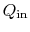and the heat rejected to the atmosphere as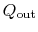. The heat out of the gas turbine is denoted as. The hot exhaust gases from the gas turbine pass through a heat exchanger where they are used as the heat source for the two-phase Rankine cycle, so thatis also the heat input to the steam cycle. The overall combined cycle efficiency is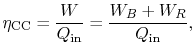where the subscripts refer to combined cycle (CC), Brayton cycle (B) and Rankine cycle (R) respectively.

From the first law, the overall efficiency can be expressed in terms of the heat inputs and heat rejections of the two cycles as (using the quantity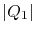to denote the magnitude of the heat transferred):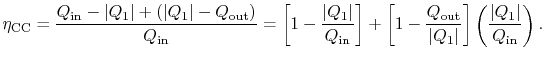The first square bracket term on the right hand side is the Brayton cycle efficiency,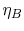, the second is the Rankine cycle efficiency,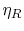, and the term in parentheses is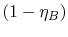. The combined cycle efficiency can thus be written as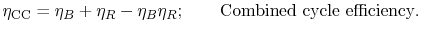(8..5)

Equation (8.5) gives insight into why combined cycles are so successful. Suppose that the gas turbine cycle has an efficiency of 40%, which is a representative value for current Brayton cycle gas turbines, and the Rankine cycle has an efficiency of 30%. The combined cycle efficiency would be 58%, which is a very large increase over either of the two simple cycles. Some representative efficiencies and power outputs for different cycles are shown in Figure 8.20.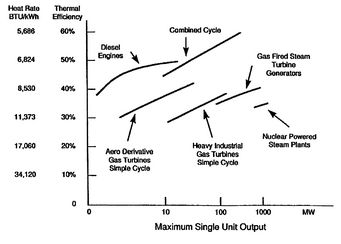UnifiedTP